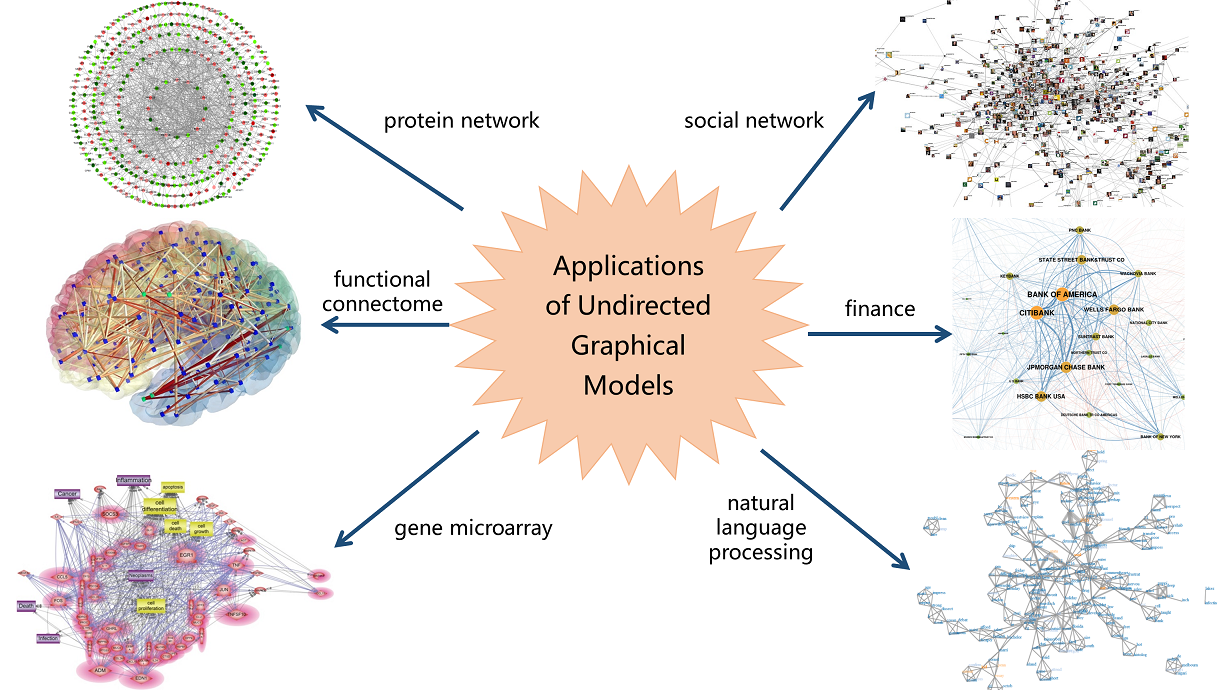# gif: Graphical Independence Filtering for Learning Large-Scale Sparse Graphical Models

## Introduction

One of the fundamental problems in data mining and statistical analysis is to detect the relationships among a set of variables. To this end, researchers apply undirected graphical models in work, which combine graph theory and probability theory to create networks that model complex probabilistic relationships. By estimating the underlying graphical model, one can capture the direct dependence between variables. In the last few decades, undirected graphical models have attracted numerous attention in various areas such as genetics, neuroscience, finance, social science and machine learning.When the data is multivariate Gaussian distributed, detecting the graphical model is equivalent to estimating the inverse covariance matrix. gif package provides efficient solutions for this problem. The core functions in gif package are hgt and sgt.

These functions based on graphical independence filtering have several advantages:

• It’s applicable to high-dimensional multivariate data and is comparable to or better than the state-of-the-art methods in respect to both graph structure recovery and parameter estimation.

• The program is very efficient and can provide solutions for problem with over 10,000 variables in less than one minute. The following table shows the time comparison of gif functions and other efficient approaches.

Method \(p = 1000\) \(p = 4000\) \(p = 10000\)
hgt 0.395s 6.668s 46.993s
sgt 0.225s 3.099s 21.454s
QUIC 1.580s 117.041s 1945.648s
fastclime 62.704s *** ***
• It has comparable performance when dealing with continuous data under various distributions though it was designed for multivariate Gaussian distribution.

Particularly, hgt provides a solution for best subset selection problem in Gaussian graphical models and sgt offers closed-form solution equivalent to graphical lasso when the graph structure is acyclic.

## Installation

### CRAN version

To install the gif R package from CRAN, just run:

``install.packages("gif")``

### Github version

To install the development version from Github, run:

``````library(devtools)
install_github("Mamba413/gif/R-package", build_vignettes = TRUE)``````

Windows user will need to install Rtools first.

## Usage

Take a synthetic dataset as a simple example to illustrate how to use hgt and sgt to estimate the underlying graphical model.

### Simulated Data

Using the function ggm.generator, we extract 200 samples from the graphical model with \(p = 100\) and whose graph structure is the so-called AR(1). A sketch of the example could be seen in the following picture.

``````set.seed(1)
n <- 200
p <- 100
Omega <- diag(1, p, p)
for(i in 1:(p - 1)) {
Omega[i, i + 1] <- 0.5
Omega[i + 1, i] <- 0.5
}
x <- ggm.generator(n, Omega)``````